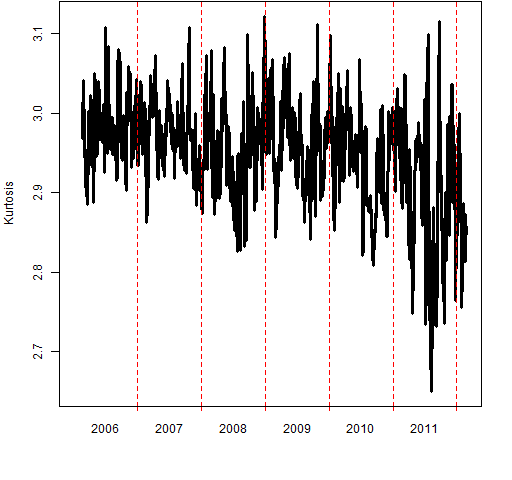Want to share your content on R-bloggers? click here if you have a blog, or here if you don't.

Not quite expected behavior of skewness and kurtosis.

## The question

In each time period the returns of a universe of stocks will have some distribution — distributions as displayed in “Replacing market indices” and Figure 1.

Figure 1: A cross-sectional distribution of simple returns of stocks.In particular they will have values for skewness and kurtosis.  When we aggregate stocks into portfolios, we would expect the cross-sectional distribution of the portfolios to be closer to the normal distribution.  That is, we expect the skewness to be closer to zero, and kurtosis to be closer to 3.

Will our expectations be met?

## Ingredients

The basic data are daily prices of almost all of the S&P 500 stocks from the start of 2006 to late February 2012.

Two sets of random portfolios were produced from this universe.  The constraints were:

• long-only
• number of names either 20 or 200
• maximum weight (10% for 20 names, 2% for 200 names)
• minimum weight (1% for 20 names, 0.1% for 200 names)

These were created as of the start of 2006 and not rebalanced.  Hence the range of weights widens as time marches on.  There were 10,000 portfolios in each set — more than necessary, but not much of a computational burden.

The skewness and kurtosis are for log returns (rather than simple returns).

## Skewness

Figure 2 shows the 200-day rolling skewness for the stock universe and the two sets of portfolios.

Figure 2: 200-day rolling skewness of log returns.The 200-name portfolios have fairly small skewness.  The expectation that the skewness of the  20-name portfolios would be smaller than the stock skewness is not always met.  Early 2011 (dates are at the end of the 200 trading days) is a particularly interesting time.  The 200-name portfolios even have bigger skewness then than the stocks.

If we switch to 30-day returns — as in Figure 3 — then it appears that the anomalous time is in mid 2010.

## Kurtosis

Figure 4 shows 200-day rolling kurtosis.  Here expectations are more forthcoming.

Figure 4: 200-day rolling kurtosis of log returns.We see the early 2011 anomaly again with only the 20-name portfolios.  The 30-day returns in Figure 5 suggest again that the real issue is in mid-2010.

Figure 5: 30-day rolling kurtosis of log returns.Figure 6 shows that the kurtosis of the 200-name portfolios is remarkably well-behaved.

Figure 6: 30-day rolling kurtosis of the log returns of the 200-name portfolios.## Summary

If you have a 200-name portfolio, then returns among 200-name portfolios you might hold will have close to a normal distribution.  Note this is not talking about the distribution of your returns through time.

If you have a 20-name portfolio, then you could have returns that look very different than those of other 20-name portfolios in the same universe.

It seems that skewness and kurtosis of portfolios can come unstuck from the skewness and kurtosis of the underlying universe.  Any ideas why?

## Appendix R

The skewness and kurtosis functions are in kurtskew.R.  This is a revised version of the file — previously the functions had a bug (of not properly centering the data).

The plot function is available at pp.timeplot.R.

#### generate random portfolios

The 20-name portfolios were generated by:

```> require(PortfolioProbe)
> rp20.sp5 <- random.portfolio(1e4, sp5.close[1,],
+    gross=1e7, long.only=TRUE, port.size=c(20,20),
+    max.weight=.1, threshold=1e7 * .01/sp5.close[1,])```

#### compute portfolio returns

```> rp20.sp5.ret <- valuation(rp20.sp5, sp5.close,
+    returns='log')```

The `sp5.close` object is the matrix of prices of all the stocks for the full time period.

#### estimate rolling skewness and kurtosis

```> rp20.skew200 <- rp20.sp5.ret[,1]
> rp20.skew200[] <- NA
> rp20.kurt200 <- rp20.skew200
> for(i in 200:length(rp20.skew200)) {
+    rp20.skew200[i] <- pp.skew(colSums(rp20.sp5.ret[
+       seq(to=i, length=200),]))
+    rp20.kurt200[i] <- pp.kurtosis(colSums(rp20.sp5.ret[
+       seq(to=i, length=200),]))
+ }```

Subscribe to the Portfolio Probe blog by Email